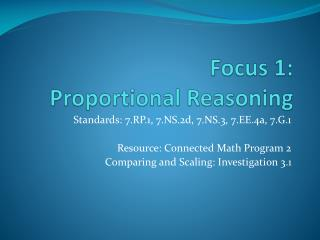DownloadDownload PresentationFocus 1: Proportional Reasoning

# Focus 1: Proportional Reasoning

Télécharger la présentation## Focus 1: Proportional Reasoning

- - - - - - - - - - - - - - - - - - - - - - - - - - - E N D - - - - - - - - - - - - - - - - - - - - - - - - - - -
##### Presentation Transcript

1. Focus 1: Proportional Reasoning Standards: 7.RP.1, 7.NS.2d, 7.NS.3, 7.EE.4a, 7.G.1 Resource: Connected Math Program 2 Comparing and Scaling: Investigation 3.1

2. Comparing and Scaling Ratio, Proportion, and Percent Investigation #3- CMP2

3. Mathematical & Problem-SolvingLearning Goals for Comparing & Scaling Rates • Examine & Connect the idea of unit rate to what you already know about ratios and about linear relationships (3.1) • Further develop understanding of unit rates and how to compute and interpret them • Work with the important application of rates to miles per hour (speed) • Introduce the concept of “average” or “steady” rate of progress • Introduce and formalize the meaning of unit rate and computation strategies for computing unit rates • Relate unit rate to the slope of the line representing the equation of the underlying relationship • Confront the issue of what it means to divide in rate situations

4. Investigation 3.1Learning Target Examine and connect the idea of unit rates to what students already know about ratios and about linear relationships

5. Investigation #3.1Comparing and Scaling Rates The following examples illustrate situations involving another strategy to compare numbers. • My mom’s car gets 45 miles per gallon on the expressway. • We need two sandwiches for each person at the picnic. • I earn \$3.50 per hour baby-sitting for my neighbor. • The mystery meat label say 355 Calories per 6-ounce serving. • My brother’s top running rate is 8.4 kilometers per hour.

6. Getting Ready for Problem 3.1 • What two quantities are being compared in the rate statements? • Which of the rate statements is different from the others? • In which of the situations is a quantity being compared to one unit of another? These are examples of rates that are called unit rates. A unit rate tells us how many per unit. Miles per hour tells how many miles are matched with 1 hour of travel and so on.

7. What are some other rates that you have encountered? • Does the statement “440 miles traveled on 20 gallons of gas” represent a unit rate? • Can you find a related unit rate? • How did you find that? • Did anyone have a different way to think about this? Now let’s use this kind of thinking to help solve the challenge of buying calculators. 

8. Vocabulary Term- RATE • Each of these statements compares two different quantities. A comparison of two quantities measured in DIFFERENT UNITS is a RATE. For example, one compares miles to gallons of gas.

9. 3.1 Technology on Sale Stores, catalogs, and Web sites often use rates in their ads. The ads sometimes give the cost for several items. You might see an offer like the one shown at the right. The listed prices are for orders of 10, 15, or 20 calculators. But it’s possible to figure the price for any number you want to purchase. One way to figure those prices is to build a rate table.

10. Problem 3.1Making and Using a Rate Table Suppose you take orders over the phone for the calculator company. You should be quick with price quotes for orders of different sizes. A. Build a rate table like the one below. Fill in prices for each type of calculator for orders of the sizes shown.

11. Use your rate table to answer questions in your Math Workbook B. How much does it cost to buy 53 fraction calculators? How much to buy 27 scientific calculators? How much to buy 9 graphing calculators? C. How many fraction calculators can a school buy it if can spend \$390? What if the school can spend only \$84?

12. D. How many graphing calculators can a school buy if it can spend \$2,500? What if the school can spend only \$560? E. What arithmetic operation (+, -, x, /) do you use to find the cost per calculator? UNIT RATE!! F. Write an equation for each kind of calculator to show how to find the price for any number ordered.

13. Explore 3.1Have you thought about? • Can you find out what a single calculator will cost? If so, how? • How can you use your answer to help fill in the rate table? • Do you see any patterns in your rate table? How would you describe them? • Does the unit rate help you to write a rule that will predict the number of miles m for any number of gallows of gas g? If so, how? (m= unit rate X g)

14. Class Discussion & Sharing What patterns do you see in the tables? What would be the shape of the graphs of the data in these tables? How would the graphs be alike and different?

15. Pre-Algebra Homework A.C.E Applications, Connections, & Extensions #1, 2, 13-16 Copy your answers in your math workbook